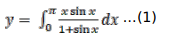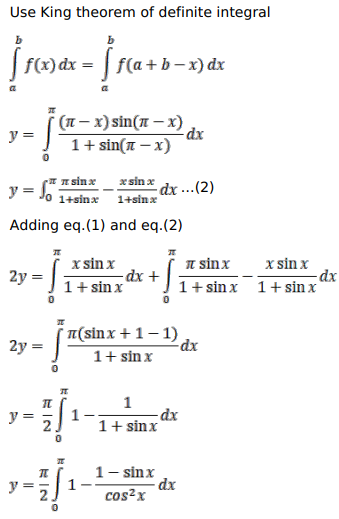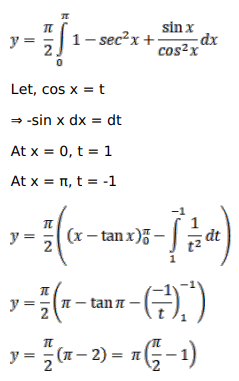# Solve this followingQuestion:

Prove that

$\int_{0}^{\pi} \frac{x \sin x}{(1+\sin x)} d x=\pi\left(\frac{\pi}{2}-1\right)$

Solution: